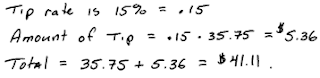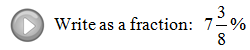## Algebra

Showing posts with label percentage. Show all posts
Showing posts with label percentage. Show all posts

### Review of Decimals and Percents

Percents are an important part of our everyday life and will show up often in our study of Algebra.  You will have to know how to convert percents to real numbers and back again.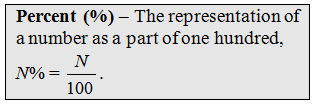Using the definition you can convert percentages to fractions very easily. Write the number over 100 and eliminate the percent symbol %, then reduce if necessary.To find a decimal equivalent divide.Notice that you could get the same result by moving the decimal over 2 places to the left.This works because a percent is part of 100 and dividing by 100 simply moves the decimal two places.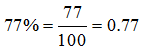Rewrite as a decimal.To write a decimal as a percent, you can multiply by 1 = 100%. For example,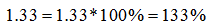This can be done quickly by moving the decimal to the right two places.  One way to remember how to convert between decimals and percents is to use the letters D and P in alphabetical order and the following illustration.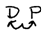If you have a decimal the illustration shows to move the decimal two places to the right. If you have a percent the illustration shows to move the decimal two places to the left.

Re-write as a percent.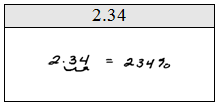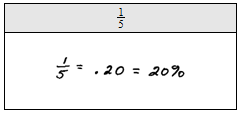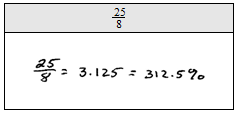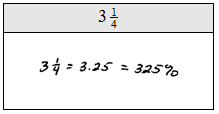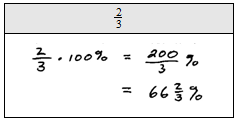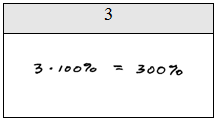When converting percents to fractions there really is no easy trick.  The idea is to use the definition and then reduce.  You must remember that a percent is a part of 100.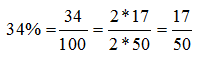Re-write as fractions.Typical Word Problems:
Tax - A calculator is advertised at \$12.99 plus a 7.5% sales tax.  What is the total including tax?Tip - After a nice dinner out, the bill came to \$35.75.  For good service you wish to leave a 15% tip.  What is the total including the tip?